Next: Deformed shell (finite non-degenerate Up: Theoretical models Previous: Degenerate shell: Seniority model

## Equidistant-level model (infinitely many levels)

Let us consider a phase space of infinitely many, doubly-degenerate, equidistant single-particle levels spaced by d. Suppose that all the levels up to a certain Fermi energy are occupied by the fermions, and that they interact through a two-body interaction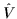. The Hamiltonian of the model reads,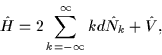(49)

where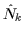is the number operator of the n-th level. Furthermore we assume thatis invariant with respect to the shift in single-particle indices:

 Vk1k2;k'1k'2 = Vk1+k,k2+k;k'1+k,k'2+k, (50)

where k is an integer number. For a moment we do not need to specify this interaction; let us only remark that the standard seniority-pairing interaction (14) obviously obeys the shift-symmetry condition.

Of course, we do not intend to consider here infinite numbers of particles and infinite energies, cf. Eq. (49). In practice, we should assume that the number of fermions interacting throughis finite but large. The remaining ones (e.g., occupying the most bound shells) form an inert core. This guarantees the effect of the finite spectrum not to be important. Consequently, in future discussions we assume that N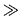1 and that the first level belonging to the interaction-active" space has k=1.

Irrespective of how complicated interactionis, its assumed shift-symmetry allows for an exact analysis in terms of filters based on ground-state energy differences. Indeed, for any particle number N, the ground-state energy reads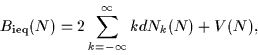(51)

where Nk(N) and V(N) are the average values of particle number operatorsand interactionin the ground-state wave functions for N particles. Since the particles interact, values of Nk(N) can be arbitrary numbers between 0 and 1; however, if we switch the interaction off (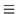0), we revert to the single-particle model (2) in which Nk=0, 0.5, or 1.

From the shift-symmetry (50), it is obvious that the change in the ground-state energy, occurring when the interaction is switched on, is the same for all even systems (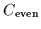) and the same for all odd systems (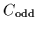) and hence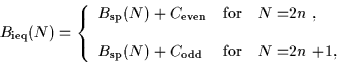(52)

where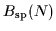is given by Eq. (2).

Filters (1), (6), and (7) now give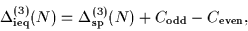(53)

hence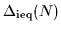=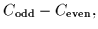(54)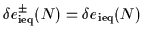= d. (55)

It is quite remarkable that the above argumentation does not at all depend on details of the two-body interaction, and that - irrespective of the interaction - filters (6) and (7) correctly separate the interaction effects (54) from the single-particle spacings (55).

This generic result does not depend on whether any approximations are used to obtain the ground-state energies of interacting systems. In particular, the BCS mean-field results (obtained for interaction) will also obey the pattern presented in Eqs. (54) exactly; only the value of the interaction-energy difference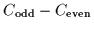may be different from the exact result.

Similarly, the BCS results obtained for the seniority-pairing interaction (14) also follow the same pattern; however, in this case we have to additionally ensure that the phase space in which the pairing correlations are allowed to develop is the same for all particle numbers. Indeed, one usually solves the BCS equations in a finite phase-space window, adjusting the interaction strength G to that window. For the results (54) to be valid, we have to always use the same number of levels below and above the Fermi level, independently of the number of particles. Actually, such a prescription is often used in realistic BCS or HFB nuclear structure calculations.

As discussed above, in the case of the equidistant-level model, the energy-filter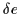reproduces the value of the level spacing dregardless of the detailed structure ofand details of the many-body approximation used. The reason for this is the shift-symmetry of the Hamiltonian. Of course, if this symmetry is broken either by assuming the finite Hilbert space (see the following section) or by introducing the explicit symmetry-violating terms, one cannot a priori expect the above conclusion to hold exactly.

It is also worth noting that the OES (54) is defined in terms of the pairing (interaction) energy in the even and odd system, and not in terms of the HFB or BCS pairing gap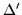(defined as half of the lowest excitation energy). Only for strong pairing correlations are the pairing gaps and the values of the OES the same (see Ref. ). In nuclei, pairing correlations never reach such a limit, and the OES and BCS pairing gap in an odd system can be significantly different.Next: Deformed shell (finite non-degenerate Up: Theoretical models Previous: Degenerate shell: Seniority model
Jacek Dobaczewski
2000-03-09